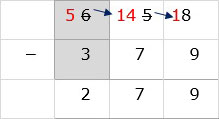# Subtraction with Multiple Regrouping Steps

#### Excel add-in with c# VSTO and web

7 Lectures 1.5 hours

#### Word add-in using c# vsto and web types

8 Lectures 1 hours

#### The Ultimate Guide To Add Social Media Login to PHP Web Apps

17 Lectures 2 hours

Subtract 836 – 647

### Solution

Step 1:

Line up the numbers vertically.Step 2:

In ones column, 7 is bigger than 6. So we borrow from 3 in tens column. We cross out 3 and write a 2. We put borrowed 1 beside 6 to make it 16. Then we subtract 7 from 16 to get 9.Step 3:

In tens column, 4 is bigger than 2. So we borrow from left. We cross out 8 and write a 7. We put borrowed 1 beside 2 to make it 12. Then we subtract 12 – 4 = 8.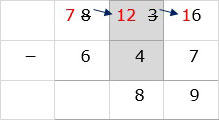Step 4:

In hundreds column, we subtract 7 – 6 = 1. So final answer is 189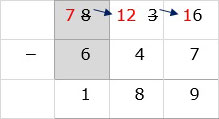Subtract 658 – 379

### Solution

Step 1:

Line up the numbers vertically.Step 2:

In ones column, 9 is bigger than 8. So we borrow from 5 in tens column. We cross out 5 and write a 4. We put borrowed 1 beside 8 to make it 18. We then subtract 18 – 9 = 9.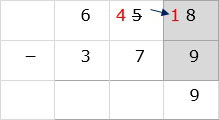Step 3:

In tens column, 7 is bigger than 4. So we borrow from left. We cross out 6 and write a 5. We put borrowed 1 beside 4 to make it 14. We then subtract 14 – 7 = 7.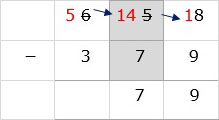Step 4:

In hundreds column, we subtract 5 – 3 = 2. So final answer is 279